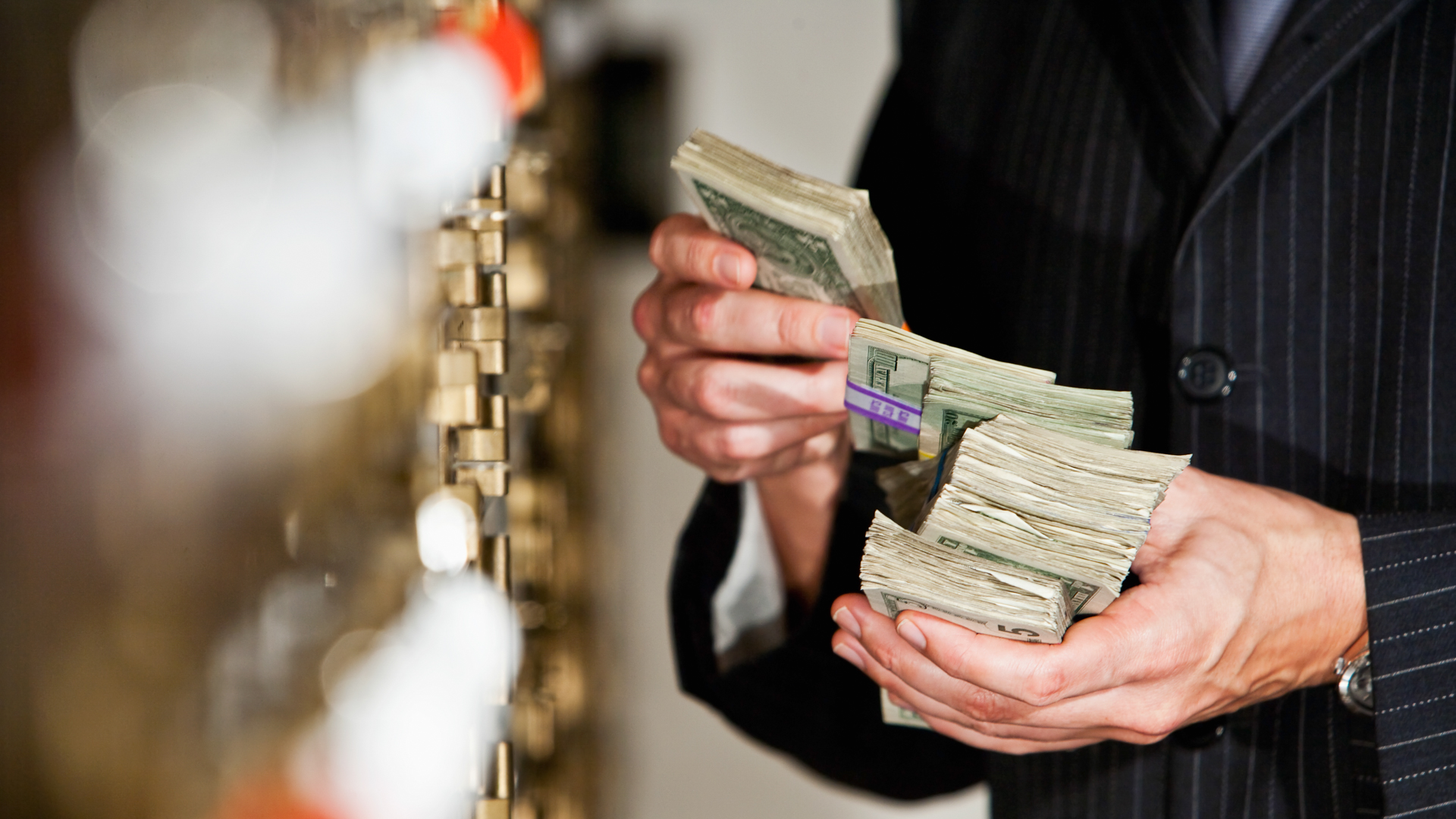# Rule of 72: See How Long It Takes To Double Your Money When Investingkali9 / iStock.com

For the climate-conscious, a marker of 72 may be good enough when you’re setting the thermostat. But when it comes to measuring money, the financially aware use lucky number 72 principally to calculate how long it will take to double their investments.

The Rule of 72 is a simple financial planning tool that can be used to calculate how fast your money will double at a given rate of return involving compound interest. Per SmartAsset, the mathematical equation is:

72 ÷ your compound annual interest rate = how many years until your investment doubles.

For example, using an annual interest rate of 8%, your dividend will double in 9 years. Conversely, if you want to know what interest rate you will need to achieve your goal of doubling your investment, reverse the equation and divide your desired number of years from 72 (72 ÷ 4 years = 18% required annual interest rate).

## Rule of 72 Has Been Around Since the 1400s

According to Wealthsimple’s Dennis Hammer, the Rule of 72 has been around for centuries. Hammer cited Italian mathematician Luca Pacioli as first referencing it in a 1494 text titled “Summary of Arithmetic, Geometry, Proportions, and Proportionality.”

“In wanting to know of any capital, at a given yearly percentage, in how many years it will double adding the interest to the capital, keep as a rule [the number] 72 in mind, which you will always divide by the interest, and what results, in that many years it will be doubled,” wrote Pacioli. “Example: When the interest is 6 percent per year, I say that one divides 72 by 6; 12 results, and in 12 years the capital will be doubled.”

Make Your Money Work Better for You

Although Pacioli didn’t enlighten his readers as to how 72 became the magic number, he did illustrate that the rule can be used to roughly calculate anything that is subject to compound interest — as long as you know the rate of growth.

## Tweaking the Rule of 72, and Applying It to Inflation

The Rule of 72 is most accurate when using an 8% interest rate and works best when interest rates fall between 6% and 10%, but the rule can be tweaked for greater accuracy if absolutely necessary. According to SmartAsset, you can modify the rule and change 72 by one number in either direction for every three points an interest rate diverges from 8% (e.g., for a rate of 11%, you would use 73; for 5%, you would use 71).

As Firstpost explains, inflation chasers can also use the Rule of 72 to find the amount of time it will take for the value of money to halve. Using its example, if the inflation rate is sitting at 7%, then the given purchasing power of money will be worth half its value in around 10.29 years (72 ÷ 7 = 10.2857143). If inflation decreases to 4%, money (without any interest being applied to combat inflationary pressure) is expected to lose half its worth in 18 years (72 ÷ 4 = 18).

More From GOBankingRates

Make Your Money Work Better for You